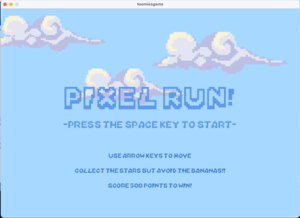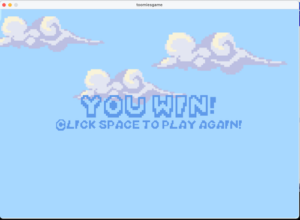# Midterm Project: Pixel Run!

For our midterm project, we were tasked with making a game!

The game I made is called Pixel Run! It’s a fun little game and I’m very proud of it!!

##### Concept

Basically, When you start the game (after you view the instructions) you will see your sprite in the center of the screen, which you can move with the arrow keys. There will be static stars at random places all around the screen and frantic bananas that move with random speeds across the screen. Your score is displayed at the top right corner of the screen.

Collect the stars!! They’re worth 20 points, but you must avoid the bananas! When your points are > 0 , they will take 10 points off your current score.

##### Goal of the game

To win, you must score 500 points! When you hit 500 or more points, you are taken to the end screen. You can press the space key to play again!

##### Different Screens

To make the different screens, I used an int variable called game mode.
I started by adding all my variables at the top of my code, then I loaded all my data like images, fonts and sounds.

I made 2 classes: one for bananas, one for stars. I made arrays for both classes and used a for loop in setup for each. I made it so that when you start the game, there are 20 stars and 10 bananas on screen.  This is also where I made the nested array for my sprite sheet. I set imageMode and textAlign to center.   I downloaded some theme music and made it loop while you play the game (The music keeps playing in all the game screens)

Here’s my setup code:

```PImage linksprite, bg, star, banana, start; // all of the images
PImage[][] sprites;
PFont mario, score;
int direction = 1;
int step = 0;
int x, y;
int r1, r2;
int speed = 4;
int gamemode = 0; // for the diff screens
int pts = 0; // points in game
import processing.sound.*;
SoundFile file;
String audioName = "music.wav";
float timer=0;
boolean timerActivated = false;

Banana[] bananas;
Star[] stars;
// arrays for both objects

void setup() {
size (1000, 700);
mario  = createFont("/Users/fatimaaljneibi/Library/Fonts/Mario-Kart-DS.ttf", 32);
score  = createFont("Courier New", 25);

//theme music loop:
file = new SoundFile(this, "music.wav");
file.loop();

stars = new Star ;
for (int i=0; i<stars.length; i++) {
stars[i]= new Star(random(width), random(height));
}

bananas = new Banana ;
for (int i=0; i<bananas.length; i++) {
bananas[i]= new Banana(random(width), random(height), random(-5, 5), random(-5, 5));
}

sprites = new PImage; // 12 images across, 4 down, in the spritesheet

for (int y=0; y < 4; y++) {
for (int x=0; x< 10; x++) {
sprites[y][x] = linksprite.get(x*w, y*h, w, h);
}
}

x = width/2;
y = height/2;

imageMode(CENTER);
textAlign(CENTER);
}```

When game mode = 0, (it starts at zero when you run the game) you can see the start screen, which looks like this:Here’s the code for the first page: (it was inside void draw)

```if (gamemode == 0) { // starting screen
background(start);
fill(#5F9AEA);
textFont(mario, 100);
text("PIXEL RUN!", width/2, height/2-25);
// instructions
textFont(mario, 40);
text("-press the space key to start-", width/2, height-300);
textFont(mario, 25);
fill(#3F7CC9);
text("use arrow keys to move", width/2, height-200);
text("collect the stars but avoid the bananas!!", width/2, height-150);
text("score 500 points to win!", width/2, height-100);

if (keyPressed) {
if (key == ' ' ) {
gamemode = 1;
pts = 0;
}
}```

I made the background on Picsart, then I downloaded a Mario Kart font and added it to my data folder. I used createFont() to use this font in my game, I used the font for the title & instructions, as well as in the win screen. When you press the space key, game mode = 1 and the next screen loads! (the actual game screen!!)

```else if (gamemode == 1) { // actual game code goes here

background(bg);

// makes the disappeared objects reappear every 5 seconds:
if (timerActivated==true) {
if (millis()>timer ) {
println("timer done!");
timerActivated = false;
for (int i=0; i<15; i++) {
if (stars[i].disp == false) {
stars[i].starx = random(width);
stars[i].stary = random(height);
}
stars[i]. disp=true;
}
for (int i=0; i<8; i++) {
bananas[i]. disp=true;
}
}
}

if (timerActivated == false && gamemode == 1) {
println("timer triggered");
timer = millis()+5000; // more objects appear on screen every 5 seconds
timerActivated = true;
}

// displays the initial stars & bananas, calls the functions from their classes
for (int i=0; i<20; i++) {
stars[i]. display();
}
for (int i=0; i<10; i++) {
bananas[i]. display();
bananas[i]. move();
}

//look at sprite sheet to determine which direction is which
if (keyPressed) {
if (keyCode == DOWN) {
direction = 0;
y+=speed;
}
if (keyCode == LEFT) {
direction = 1;
x-=speed;
}
if (keyCode == RIGHT) {
direction = 3;
x+=speed;
}
if (keyCode == UP) {
direction = 2;
y-=speed;
}
if (key == 'b' || key == 'B' ) {
gamemode = 0;
}
if (keyCode == DOWN || keyCode ==  UP || keyCode == LEFT || keyCode == RIGHT) {

if (frameCount%speed==0) { //the spritesheet images will loop only if the arrow keys are pressed
step = (step+1) % 10;
}
}
}

image(sprites[direction][step], x, y); // the sprite

// points system:
for (int i=0; i<10; i++) {
float d2 = dist(x, y, bananas[i].banx, bananas[i].bany);
if ( d2 <= 50 && bananas[i].disp==true) {
if (pts >=10)
pts-= 10;
// takes 10 points off for every banana collided w the sprite

bananas[i].disp=false; //banana disappears
}
}

for (int i=0; i<20; i++) {
float d1 = dist(x, y, stars[i].starx, stars[i].stary);
if ( d1 <= 50 && stars[i].disp==true) {
pts+= 20;
stars[i].disp=false;  //adds 20 pts per star and makes it disappear on contact w sprite
}
}

if (y>height) {
y=0;
}
if (y<0) {
y=height;
}
if (x>width) {
x=0;
}
if (x<0) {
x=width;
} // this is to keep the sprite always on screen

//the score board:
textFont(score);

pushStyle();
fill(255, 200);
noStroke();
rectMode(CENTER);
rect(width-80, 22, textWidth("Score:" + pts)+10, 30);
popStyle();
fill(0);
text("Score:" + pts, width-80, 30);
pushStyle();
fill(255, 180);
noStroke();
rect(2, height-20, 270, 15 );
textAlign(LEFT);
textFont(score, 15);
fill(0);
text("press 'b' to go back to start!", 2, height-8);
popStyle();

if (pts >= 500) {
gamemode = 3;  //game ends when the player hits 500 pts
}
} // game code ends here
```

There’s a lot to unpack here:

When gamemode = 1, the background changes, the sprite appears at the center of the screen, the stars appear at random spots(they do not move) the bananas also appear at random spots, but they move with a random speed ranging from -5 to 5. This is because I added an extra argument for the bananas, which is spx and spy (speed x and speed y).

The sprite moves in directions 0-3, corresponding to the row on the sprite sheet image.  The sprite is always on screen because of this code:

```if (y>height) {
y=0;
}
if (y<0) {
y=height;
}
if (x>width) {
x=0;
}
if (x<0) {
x=width;
} // this is to keep the sprite always on screen
```

I used a similar code in the bananas class, so that the bananas always appear on screen as well.

The bananas and the stars classes have a display() function, which displays them at random spots on screen. The banana also has a move() function.

When the sprite collides with a star or a banana, it disappears and the pts variable adds or subtracts points depending on whether it is a star or a banana. These images disappear because of a boolean disp in their classes which makes them disappear on contact with the sprite.

The way collision detection works here is it checks to see if the image is displayed (the boolean disp I mentioned earlier) and if it is && the distance between the sprite’s x and y positions and the image’s x and y positions is less than 50 pixels, the image(star or banana) will disappear.

I also made a timer which will make the disappeared objects ( if their disp boolean =false and the 5 seconds have passed, the star or banana will reappear at a different spot. I made it so that 8 bananas and 15 stars can reappear every 5 seconds. This happens continuously.

Here’s the code for the timer:

```// makes the disappeared objects reappear every 5 seconds:
if (timerActivated==true) {
if (millis()>timer ) {
println("timer done!");
timerActivated = false;
for (int i=0; i<15; i++) {
if (stars[i].disp == false) {
stars[i].starx = random(width);
stars[i].stary = random(height);
}
stars[i]. disp=true;
}
for (int i=0; i<8; i++) {
bananas[i]. disp=true;
}
}
}

if (timerActivated == false && gamemode == 1) {
println("timer triggered");
timer = millis()+5000; // more objects appear on screen every 5 seconds
timerActivated = true;```

I also added a rectangle underneath the score counter & the bottom right text, it is not fully opaque so that you can see if there are any objects in that area of the screen. I used push & pop style here to make the rectMode= center for the score one.

When you hit 500 points or more, gamemode= 3 and the win screen is displayed. It looks like this :As you can see from the image, clicking the space bar will reset the game.

Here is the code for the bananas class:

```class Banana {
float banx, bany;    // bananas position
float spx, spy;     //  speed
float banw, banh; //width & height of the image
boolean disp = true;

Banana(float _banx, float _bany, float _spx ,float _spy ) {
banx = _banx;
bany = _bany;

banw = 40;
banh= 40;

spx = _spx;
spy = _spy;

}

void display() {
if (disp)
image(banana, banx, bany, banw, banh);
// displays the bananas on screen
}

void move() {
banx += spx;
bany += spy;

if (bany>height) {
bany=0;
}
if (bany<0) {
bany=height;
}
if (banx>width) {
banx=0;
}
if (banx<0) {
banx=width;
//banana is always moving on screen
}
}
}
```

Stars class:

```class Star {
float starx, stary;    // stars position
float starw, starh; //width & height of the image
boolean disp = true;

Star(float _starx, float _stary) {
starx = _starx;
stary = _stary;

starw = 40;
starh= 40;

}

void display() {
if(disp)
image(star, starx, stary, starw, starh);
// displays the stars on screen
}

}
```

Here is the the gameplay! TA-DA!!

https://youtu.be/r99jzLBahlk

(the “press  ‘b’ to go back to start!” text is not in this recording, but its in the game code now! I added it in after I recorded this!)

Here’s the zip file for the whole game:

toomiesgame